### Smoothers

You can specify three types of smoothers in the MODEL statement:

• SPLINE(x) specifies a cubic smoothing spline term for variable x

• LOESS(x) specifies a loess term for variable x

• SPLINE2(x1, x2) specifies a thin-plate smoothing spline term for variables x1 and x2

A smoother is a tool for summarizing the trend of a response measurement Y as a function of one or more predictor measurements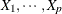. It produces an estimate of the trend that is less variable than Y itself. An important property of a smoother is its nonparametric nature. It does not assume a rigid form for the dependence of Y on. This section gives a brief overview of the smoothers that can be used with the GAM procedure.

#### Cubic Smoothing Spline

A smoothing spline is the solution to the following optimization problem: among all functions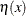with two continuous derivatives, find one that minimizes the penalized least square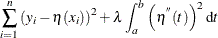whereis a fixed constant and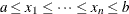. The first term measures closeness to the data while the second term penalizes curvature in the function. It can be shown that there exists an explicit, unique minimizer, and that minimizer is a natural cubic spline with knots at the unique values of.

The value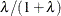is the smoothing parameter. Whenis large, the smoothing parameter is close to 1, producing a smoother curve; small values of, corresponding to smoothing parameters near 0, are apt to produce rougher curves, more nearly interpolating the data.

#### Local Regression

Local regression was proposed by Cleveland, Devlin, and Grosse (1988). The idea of local regression is that at a predictor x, the regression functioncan be locally approximated by the value of a function in some specified parametric class. Such a local approximation is obtained by fitting a regression surface to the data points within a chosen neighborhood of the point x. A weighted least squares algorithm is used to fit linear functions of the predictors at the centers of neighborhoods. The radius of each neighborhood is chosen so that the neighborhood contains a specified percentage of the data points. The smoothing parameter for the local regression procedure, which controls the smoothness of the estimated curve, is the fraction of the data in each local neighborhood. Data points in a given local neighborhood are weighted by a smooth decreasing function of their distance from the center of the neighborhood. See Chapter 53: The LOESS Procedure, for more details.

#### Thin-Plate Smoothing Spline

The thin-plate smoothing spline is a multivariate version of the cubic smoothing spline. The theoretical foundations for the thin-plate smoothing spline are described in Duchon (1976, 1977); Meinguet (1979). The smoothing parameter for the thin-plate smoothing spline smoother is the parameter that controls the smoothness penalty. When the smoothing parameter is close to 0, the fit is close to an interpolation. When the smoothing parameter is very large, the fit is a smooth surface. Further results and applications are given in Wahba and Wendelberger (1980). See Chapter 96: The TPSPLINE Procedure, for more details.